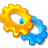# libssrckdtree-j Generic k-d tree Java librarylibssrckdtree-j is a Java class library of spatial data structures, currently containing only an implementation of a k-d tree conforming to the `java.util.Map` interface. Additional spatial data structures may be added in the future. libssrckdtree-j implements a fully generalized multi-dimensional k-d tree. You can use keys with two, three, or more dimensions whose elements implment the `Comparable` interface. Stored values may be of any type derived from `java.lang.Object`. K-nearest neighbors search is implemented as of version 1.0.1.

If you require high-performance multi-dimensional range-searching using generic data structures, you may want to consider using the libssrckdtree C++ template library instead.

 Version Source License Changes Documentation Reports 1.0.2 libssrckdtree-j-1.0.2.tar.bz2 LICENSE CHANGES 1.0.2 API Test API Test Code Coverage 1.0.1 libssrckdtree-j-1.0.1.tar.bz2 LICENSE 1.0.0 libssrckdtree-j-1.0.0.tar.bz2 LICENSE

# A Simple Example

The `KDTree` class implements the `java.util.Map` interface and adds a range-searching iterator function. The following example inserts some point/string pairs into a `KDTree` instance and iterates over a range to print some key-value pairs.

import java.util.*; import com.savarese.spatial.*; public final class kdtreedemo { public static void fillMap(Map map, Set points) { for(Object point : points) map.put(point, point.toString()); } public static HashSet generatePoints() { HashSet points = new HashSet(); Random random = new Random(); for(int i = 0; i < 100; ++i) { int x = random.nextInt(100); int y = random.nextInt(100); points.add(new GenericPoint<Integer>(new Integer(x), new Integer(y))); } return points; } public static final void main(String argv[]) { HashSet points = generatePoints(); RangeSearchTree<Integer, GenericPoint<Integer>, java.lang.String> tree = new KDTree<Integer, GenericPoint<Integer>, java.lang.String>(); fillMap(tree, points); Iterator<Map.Entry<GenericPoint<Integer>,java.lang.String>> range = tree.iterator(new GenericPoint<Integer>(25, 25), new GenericPoint<Integer>(75, 75)); while(range.hasNext()) { Map.Entry<GenericPoint<Integer>,java.lang.String> e = range.next(); System.out.println(e.getKey() + " : " + e.getValue()); } } }